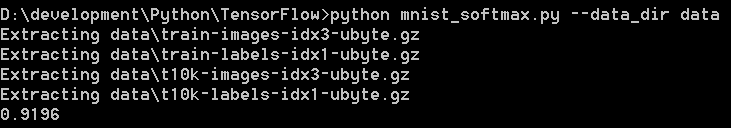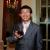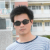Tensorflow第一个例子 原荐

Anaconda_PATH\envs\TensorFlow\Lib\site-packages\tensorflow\examples\tutorials\mnist

mnist_softmax.py文件的内容如下：

#
# Licensed under the Apache License, Version 2.0 (the "License");
# you may not use this file except in compliance with the License.
# You may obtain a copy of the License at
#
#
# Unless required by applicable law or agreed to in writing, software
# distributed under the License is distributed on an "AS IS" BASIS,
# WITHOUT WARRANTIES OR CONDITIONS OF ANY KIND, either express or implied.
# See the License for the specific language governing permissions and
# limitations under the License.
# ==============================================================================

"""A very simple MNIST classifier.

See extensive documentation at
http://tensorflow.org/tutorials/mnist/beginners/index.md
"""
from __future__ import absolute_import
from __future__ import division
from __future__ import print_function

import argparse
import sys

# Import data
from tensorflow.examples.tutorials.mnist import input_data

import tensorflow as tf

FLAGS = None

def main(_):
mnist = input_data.read_data_sets(FLAGS.data_dir, one_hot=True)

# Create the model
x = tf.placeholder(tf.float32, [None, 784])
W = tf.Variable(tf.zeros([784, 10]))
b = tf.Variable(tf.zeros())
y = tf.matmul(x, W) + b

# Define loss and optimizer
y_ = tf.placeholder(tf.float32, [None, 10])

# The raw formulation of cross-entropy,
#
#   tf.reduce_mean(-tf.reduce_sum(y_ * tf.log(tf.nn.softmax(y)),
#                                 reduction_indices=))
#
# can be numerically unstable.
#
# So here we use tf.nn.softmax_cross_entropy_with_logits on the raw
# outputs of 'y', and then average across the batch.
cross_entropy = tf.reduce_mean(tf.nn.softmax_cross_entropy_with_logits(y, y_))

sess = tf.InteractiveSession()
# Train
tf.global_variables_initializer().run()
for _ in range(1000):
batch_xs, batch_ys = mnist.train.next_batch(100)
sess.run(train_step, feed_dict={x: batch_xs, y_: batch_ys})

# Test trained model
correct_prediction = tf.equal(tf.argmax(y, 1), tf.argmax(y_, 1))
accuracy = tf.reduce_mean(tf.cast(correct_prediction, tf.float32))
print(sess.run(accuracy, feed_dict={x: mnist.test.images,
y_: mnist.test.labels}))

if __name__ == '__main__':
parser = argparse.ArgumentParser()
help='Directory for storing input data')
FLAGS, unparsed = parser.parse_known_args()
tf.app.run(main=main, argv=[sys.argv] + unparsed)

python mnist_softmax.py

http://yann.lecun.com/exdb/mnist/train-images-idx3-ubyte.gz

http://yann.lecun.com/exdb/mnist/train-labels-idx1-ubyte.gz

http://yann.lecun.com/exdb/mnist/t10k-images-idx3-ubyte.gz

http://yann.lecun.com/exdb/mnist/t10k-labels-idx1-ubyte.gz

python mnist_softmax.py --data_dir datafrom tensorflow.examples.tutorials.mnist import input_data
mnist = input_data.read_data_sets(FLAGS.data_dir, one_hot=True)

import tensorflow as tf

x = tf.placeholder(tf.float32, [None, 784])

W = tf.Variable(tf.zeros([784, 10]))
b = tf.Variable(tf.zeros())

y = tf.nn.softmax(tf.matmul(x, W) + b)

y = tf.matmul(x, W) + b

y_ = tf.placeholder(tf.float32, [None, 10])

cross_entropy = tf.reduce_mean(tf.nn.softmax_cross_entropy_with_logits(y, y_))

cross_entropy = - sum_i y'_i log(y_i)

y' 代表每张图所代表的真实值，比如 3 的话，就是（0，0，0，1，0，0，0，0，0，0）。交叉熵越大，意味着结果越差。因此第2行定义了y_占位符来保存真正的输出值，第3行给出了交叉熵的定义。

https://www.tensorflow.org/api_guides/python/train#optimizers

sess = tf.InteractiveSession()

tf.global_variables_initializer().run()
for _ in range(1000):
batch_xs, batch_ys = mnist.train.next_batch(100)
sess.run(train_step, feed_dict={x: batch_xs, y_: batch_ys})

correct_prediction = tf.equal(tf.argmax(y, 1), tf.argmax(y_, 1))
accuracy = tf.reduce_mean(tf.cast(correct_prediction, tf.float32))
print(sess.run(accuracy, feed_dict={x: mnist.test.images,
y_: mnist.test.labels}))

tf.argmax函数给出张量沿某个方向的最大值所在的指标，因此tf.argmax(y,1)给出神经网络模型所认为的图片对应的数字，而tf.argmax(y_,1)则给出该图片真实对应的数字。correct_prediction表示神经网络模型预言是否正确。第2行则将这些对错序列转化为浮点数，比如[true, true, false, true,...]转化为[1,1,0,1,...]，然后计算其平均值。最后一行将最终的正确率打印出来。如前面图中的结果所示，正确率达到0.9196，约为92%，还算不错。

https://www.tensorflow.org/get_started/mnist/beginners评论(4)引用来自“Yashin”的评论

“在此例中，采用了单层10个神经元的神经网络” TensorFlow 是如何指定神经元的层数和个数的呢？还是说神经网络对代码来说是透明的？更高级的例子中神经元之间的调用在TensorFlow中是否也是透明的？昨天大概看了一下TensorFlow发现不太理解，望不吝赐教😆

引用来自“Yashin”的评论

https://my.oschina.net/propagator/blog/856728引用来自“Yashin”的评论

“在此例中，采用了单层10个神经元的神经网络” TensorFlow 是如何指定神经元的层数和个数的呢？还是说神经网络对代码来说是透明的？更高级的例子中神经元之间的调用在TensorFlow中是否也是透明的？昨天大概看了一下TensorFlow发现不太理解，望不吝赐教😆

引用来自“propagator”的评论引用来自“Yashin”的评论

“在此例中，采用了单层10个神经元的神经网络” TensorFlow 是如何指定神经元的层数和个数的呢？还是说神经网络对代码来说是透明的？更高级的例子中神经元之间的调用在TensorFlow中是否也是透明的？昨天大概看了一下TensorFlow发现不太理解，望不吝赐教😆“在此例中，采用了单层10个神经元的神经网络” TensorFlow 是如何指定神经元的层数和个数的呢？还是说神经网络对代码来说是透明的？更高级的例子中神经元之间的调用在TensorFlow中是否也是透明的？昨天大概看了一下TensorFlow发现不太理解，望不吝赐教😆
tensorflow 获取变量&打印权值等方法

tensorflow 获取变量&打印权值等方法 在使用tensorflow中，我们常常需要获取某个变量的值，比如：打印某一层的权重，通常我们可以直接利用变量的name属性 来获取，但是当我们利用一些第三方的...

cassiepython
2018/01/27
0
0
【翻译】Sklearn与TensorFlow机器学习实用指南 ——第12章 设备和服务器上的分布式TensorFlow（下）

2018/06/29
0
0

TensorFlow 2.0测试版在今年春季发布，新版本比1.x版本在易用性上有了很大的提升。但是由于2.0发布还没有多久，现在大部分论文的实现代码都是1.x版本的，所以在学习TensorFlow的过程中同时安...

08/02
0
0

2018/05/31
0
0
TensorFlow从1到2（一）续讲从锅炉工到AI专家

04/07
0
0

OSChina 周一乱弹 —— 人生，还真是到处是意外

Osc乱弹歌单（2019）请戳（这里） 【今日歌曲】 @这次装个文艺青年吧 ：#今日歌曲推荐# 分享lil peep的单曲《High School》 《High School》- lil peep 手机党少年们想听歌，请使劲儿戳（这里...

46分钟前
19
2

CREATE_17

6
0
CSS盒子模型

CSS盒子模型 组成： content --> padding --> border --> margin 像现实生活中的快递： 物品 --> 填充物 --> 包装盒 --> 盒子与盒子之间的间距 content ：width、height组成的 内容区域 padd......

studywin

7
0

locbytes

10
0
jquery 添加和删除节点

8
0